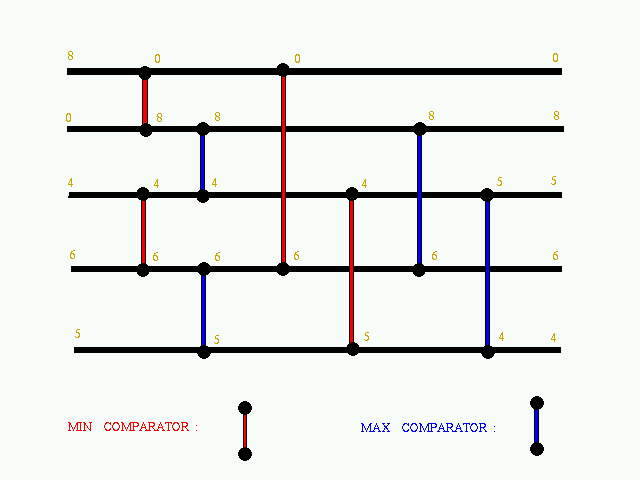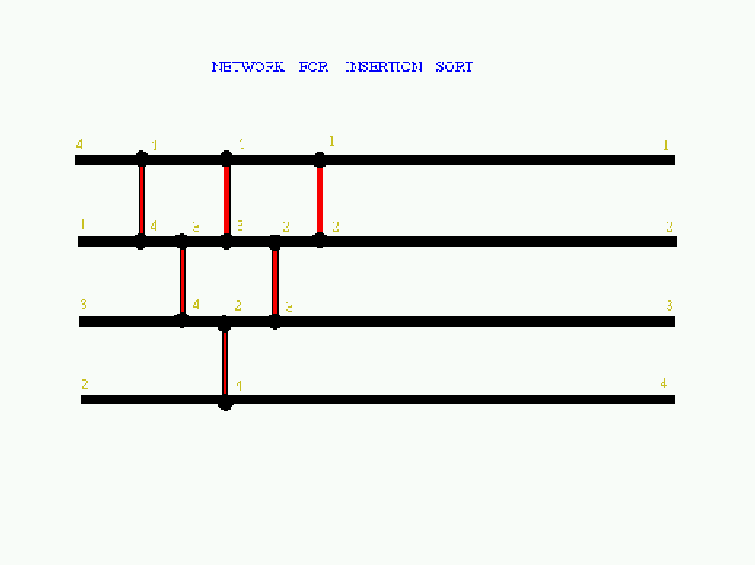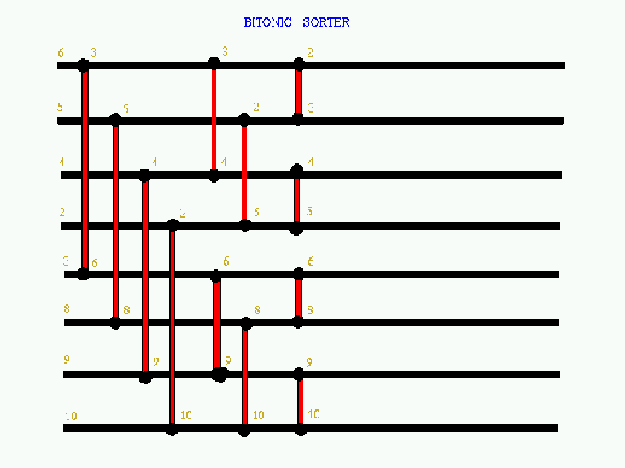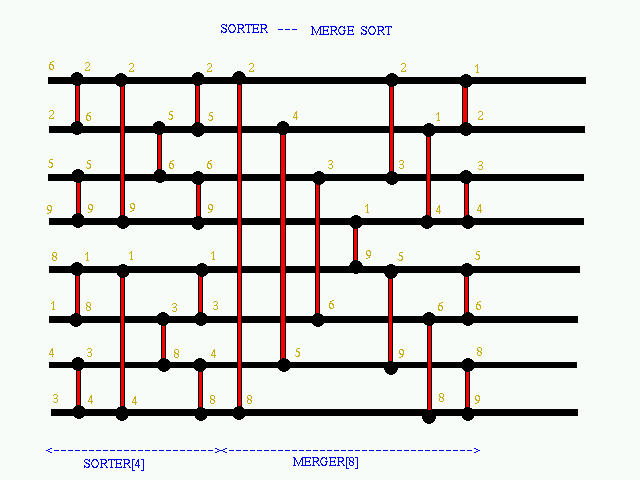Department of Computer Science and Engineering, IIT Kanpur

## CS210: Data Structures

### Dr. R. K. Ghosh

Home | Notice Board | Instructor | TAs | Students | Assignments | Schedule | Lectures | Resources
Sorting Network

 A comparison network is a network consistng of wires and connections between the lines known as comparators. As an example, picture of a simple network is provided belowTwo types of comparators are possible: min comparator max comparator A min comparator is one which takes as input two data points,say x1 and x2 on its upper end and lower end respectively,and produces output as min(x1,x2) and max(x1,x2) on its upper end and lower end respectively. A max comparator is just the opposite of the min comparator in the sense that maximum is produced at the upper end and the minimum is produced at the lower end. Through comparators and wires we can design a complete network which can produce results according to the input and the placement of the comparators in between them. The two comparators are said to be in parallel if they get both its input simultaneously so that they can produce output simultaneously.All the comparators which are in parallel require one unit of time to give output once they have got both the inputs. Hence ,in effect, the whole network takes 'n' unit of time to produce final output after being given the data where n is equal to the no. of parallel levels in the network. Various things are possible on this network. We can design our own circuit and get outputs according to that. Circuit for varous sorting are possible such as:
Insertion sortBitonic sorterHalf cleaner, Merger, SorterThe 0-1 principle

 Also we can decide whether the given network is sorting or not. This can be checked by what we call as the famous 0-1 principle. This principle states that a given network is sorting iff (if and only if) it sorts all the 2^n combination of 0 and 1 as input. This principle can be proved with the help of the fact that if the input to the network is a sequence and its output is and  'f' is a monotonically non-decreasing function then the input will have the output as . So this principle can be applied to determine whether the given network is sorting or not. It starts from <0,0,............,0> as input and go till <1,1,.................,1> and stops as soon as it discover that the ouptut is not sorted.If the output is sorted for all values till last then the network is sorting.

Home | Notice Board | Instructor | TAs | Students | Assignments | Schedule | Lectures | Resources